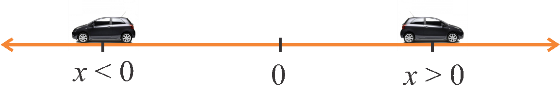In the verge of coronavirus pandemic, we are providing FREE access to our entire Online Curriculum to ensure Learning Doesn't STOP!

# Labelling Points on a Number Line

Go back to  'Coordinate-Geometry'

Suppose that a car is traveling on a perfectly straight line, and it can move either forward or backward. At any given instant, how can its position be described?

This is easy. We just create a reference position, and call it our zero. We assign to it the number 0. If the car is exactly at this point, we will say that its position is x = 0 (of course, the entire car cannot be at this position at the same time - but we are just having a hypothetical discussion; so let us decide that when we say that the car is at some position, we mean to say that, for example, the midpoint of the base of the car is on top of that position).

Now, if at some given instant, the car is to the right of 0, it can be assigned a positive real number x$$>$$ 0. On the other hand, if the car is to the left of 0, it can be assigned a negative real number x $$<$$ 0, as shown below:Thus the position of the car is defined uniquely at any given instant by exactly one real number. If you are given the position (real number) of the car at any instant, you can immediately plot the position of the car on the line, that is, pinpoint the position at which the car is at that instant. For example, if someone says that at a particular instant, the car is at x = 21.314 m, you can immediately pinpoint the exact location of the car at that instant.We will say this fact in the following manner: any position on a straight line can be labeled by exactly one real number.

Coordinate Geometry
Grade 9 | Questions Set 1
Coordinate Geometry
Coordinate Geometry
Grade 10 | Questions Set 1
Coordinate Geometry